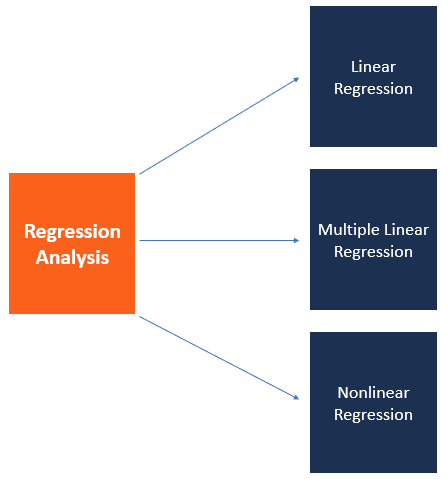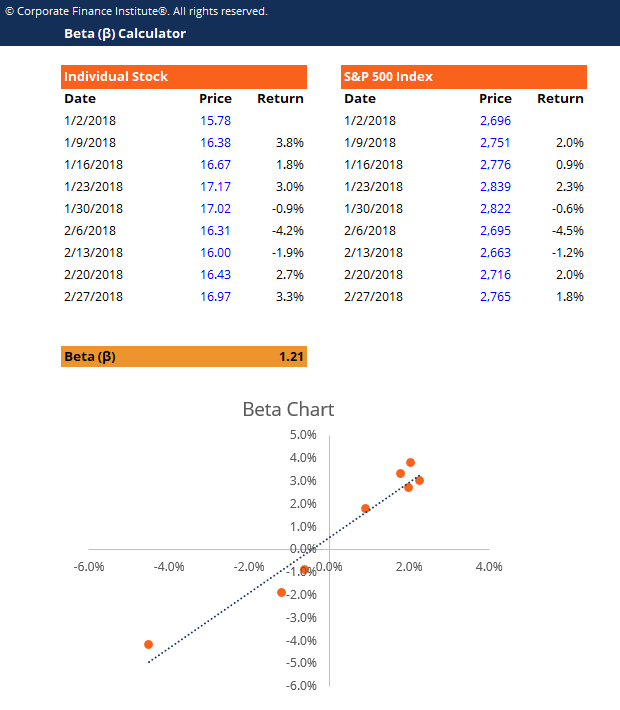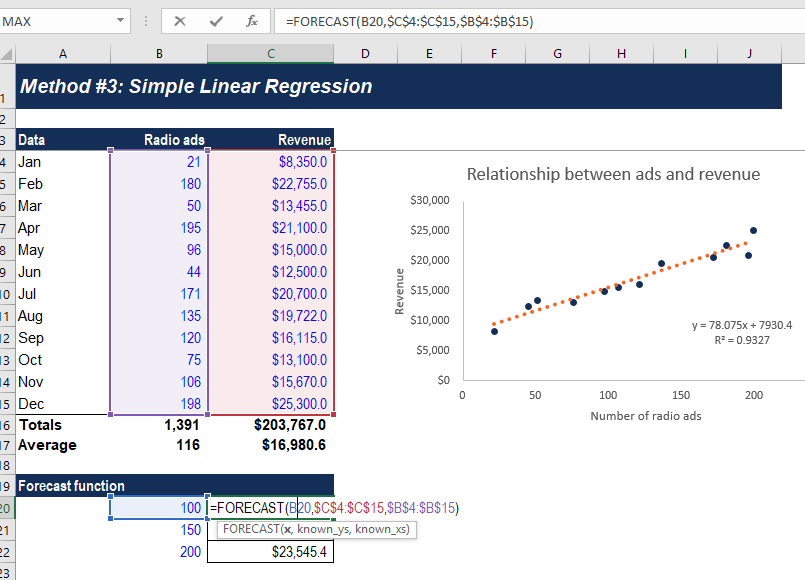# Regression Analysis

The estimation of relationships between a dependent variable and one or more independent variables

## What is Regression Analysis?

Regression analysis is a set of statistical methods used for the estimation of relationships between a dependent variable and one or more independent variables. It can be utilized to assess the strength of the relationship between variables and for modeling the future relationship between them.Regression analysis includes several variations, such as linear, multiple linear, and nonlinear. The most common models are simple linear and multiple linear. Nonlinear regression analysis is commonly used for more complicated data sets in which the dependent and independent variables show a nonlinear relationship.

Regression analysis offers numerous applications in various disciplines, including finance.

### Regression Analysis – Linear model assumptions

Linear regression analysis is based on six fundamental assumptions:

1. The dependent and independent variables show a linear relationship between the slope and the intercept.
2. The independent variable is not random.
3. The value of the residual (error) is zero.
4. The value of the residual (error) is constant across all observations.
5. The value of the residual (error) is not correlated across all observations.
6. The residual (error) values follow the normal distribution.

### Regression Analysis – Simple linear regression

Simple linear regression is a model that assesses the relationship between a dependent variable and one independent variable. The simple linear model is expressed using the following equation:

##### Y = a + bX + ϵ

Where:

Y – dependent variable

X – independent (explanatory) variable

a – intercept

b – slope

ϵ – residual (error)

### Regression Analysis – Multiple linear regression

Multiple linear regression analysis is essentially similar to the simple linear model, with the exception that multiple independent variables are used in the model. The mathematical representation of multiple linear regression is:

##### Y = a + bX1 + cX2 + dX3 + ϵ

Where:

Y – dependent variable

X1, X2, X– independent (explanatory) variables

a – intercept

b, c, d – slopes

ϵ – residual (error)

Multiple linear regression follows the same conditions as the simple linear model. However, since there are several independent variables in multiple linear analysis, there is another mandatory condition for the model:

• Non-collinearity: Independent variables should show a minimum of correlation with each other. If the independent variables are highly correlated with each other, it will be difficult to assess the true relationships between the dependent and independent variables.

### Regression analysis in finance

Regression analysis has several applications in finance. For example, the statistical method is fundamental to the Capital Asset Pricing Model (CAPM). Essentially, the CAPM equation is a model that determines the relationship between the expected return of an asset and the market risk premium.

The analysis is also used to forecast the returns of securities based on different factors, or forecast the performance of a business. Learn more forecasting methods in CFI’s Budgeting and Forecasting Course!

#### 1. Beta and CAPM

In finance, regression analysis is used to calculate the Beta (volatility of returns relative to the market) for a stock. It can be done in Excel using the Slope function.#### 2. Forecasting Revenues and Expenses

When forecasting financial statements for a company, it may be useful to do a multiple regression analysis to determine how changes in certain assumptions or drivers of the business will impact revenue or expenses in the future. For example, there may be a very high correlation between the number of salespeople employed by a company, the number of stores they operate, and the revenue the business generates.The above example shows how to use the Forecast function in Excel to calculate a company’s revenue based on the number of ads it runs.

We hope you’ve enjoyed reading CFI’s explanation of regression analysis. CFI offers the Financial Modeling & Valuation Analyst (FMVA)™ certification program for those looking to take their careers to the next level. To learn more about related topics, check out the following resources:

• Cost Behavior Analysis
• Financial Modeling Skills
• Forecasting Methods
• High-Low Method

### Financial Analyst Training

Get world-class financial training with CFI’s online certified financial analyst training program!

Gain the confidence you need to move up the ladder in a high powered corporate finance career path.

Learn financial modeling and valuation in Excel the easy way, with step-by-step training.